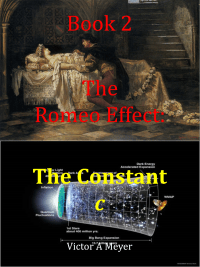# Book 2 – The Constant cAuthor:  Victor A Meyer
Last Version:  Unwritten
# of Words:  Unknown
Writing Status:    Planning Stages
Publication Status:    Unpublished

This second book in The Romeo Effect series corrects our understanding of the constant c. In the Theory of Relativity, c stands for the speed of light in the vacuum of space. Under the Theory of Time, c represents the speed our universe moving in the Spatial-Time dimension – a fourth spatial dimension in the Multiverse. The mass-energy equivalence equation E=mc2 is an acceleration formula where matter was accelerated to speed c at the time our universe was created.

## Proposed List of Chapters

Chapter 1 – Romeo Effect, Non-Constant Light Speed, and the Spatial-Time Dimension:

Chapter 2 – The Current Interpretation of the Constant c:

Chapter 3 – The Lorentz Factor and Time Dilation:

Chapter 4 – Entropy and Thermodynamics:

Chapter 5 – Extending Einstein’s Ideas to the Spatial-Time Dimension:

Chapter 6 – Einstein’s Energy-Mass Equivalence Equation:

Chapter 7 – A New Interpretation of the Constant c:

Chapter 8 – The Composite and Spatial-Time Dimensions:

Chapter 9 – Dual Universes:

## Theses Discussed in Book

Thesis #1 – The speed of light is non-constant

Thesis #2 – Time dilation only exists in clocks

Thesis #4 – Anything can travel faster than c

Thesis #6 – Spatial dimensions are Euclidean

Thesis #7 – There exists a fourth Spatial-Time Dimension in the Multiverse

Thesis #8 – Einstein’s Mass-Energy Equivalence formula is an acceleration formula

Thesis #9 – The constant c is a universal constant representing the speed of matter in the Spatial-Time Dimension

Thesis #10 – The Lorentz Factor, γ, is the conversion factor between cL and cT

Thesis #11 – There exists two dimensions of time – one with speed zero and one with speed cT

Thesis #12 – Experiments that measure cL are measuring cT

Thesis #13 – The speed of light is dependent on the relative velocity of the light source

Thesis #15 – Entropy is higher degrees-of-freedom governed by temperature

Thesis #16 – Our universe follows an Inverse Cube Law based on entropy

Thesis #17 – The frequency, f, of a photon decreases over time due to entropy

Thesis #18 – The wavelength, λ, of a photon changes as the speed of the photon changes

Thesis #24 – The CMB is an example of Entropic Redshift over time

Thesis #26 – The Spatial-Time Dimension had a beginning

Thesis #27 – An Arrow of Time is based on movement along the Spatial-Time Dimension

Thesis #28 – All universes are born as twins

Thesis #29 –Time-reversal means following the opposite Arrow of Time

Thesis #30 – There is no Baryogenesis

Thesis #31 – Particles are waveforms travelling at speed cT